free newsletter!tell a friend!contestssoftware
reviews
bestseller list
price survey
what's new
product support
searcheducational tools
math worksheets
vocabulary builders
hangman
logic games
brain foodeducational insightsfeature articlesmarketplace
movie cornerSuperKids home
humor
help* * *

Promotions

* * *math worksheets > > fractions > > subtracting fractions

## SuperKids Math Review

### How to Subtract Fractions

 Remember . . .Here's a memory trick: the Denominator is the bottom, or Down number in a fraction -- and both Denominator and Down start with the letter D.

Subtracting Fractions with COMMON Denominators
Subtracting fractions with COMMON denominators is simple. Just subtract the top numbers (the numerators), and place the resulting answer in the top of a fraction using the existing denominator for the bottom number. Then reduce the fraction, if possible

Example 1: Simple fraction subtraction-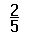=No reduction is possible, so we have found the answer!

Example 2: Reducing the fraction answer-=Then reduce:=Example 3: Subtraction with a mixed number-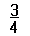= ?

First convert the mixed number to an improper fraction:=Then subtract:-=Then reduce the fraction:=Creating Common Denominators How do we do that? Simple! Remember, if you multiply the top and bottom of a fraction by the same number, it doesn't affect the value of the fraction. Example 1: If we have the fraction 2/3, we can multiply the top and bottom by 2, and not change its value: (2/2) x (2/3) = 4/6 Then if we reduce 4/6, we still get the original number, 2/3 Example 2: If we have the fraction 2/3, we could multiply top and bottom by 5, and not change its value: (5/5) x (2/3) = 10/15. Then if we reduce 10/15, we still get the original number, 2/3. Why does this work? Because any number divided by itself equals one. 2/2 = 1, 5/5 = 1, etc. And any number multiplied by 1 equals itself! The point is, you don't change the value of a fraction if you multiply its top and bottom numbers by the same number!

Subtracting Fractions with DIFFERENT denominators
You can only subtract fractions which have the same denominator, so you must first change one or both of the fractions to end up with two fractions having a common denominator. The easiest way to do this, is to simply select the opposite fraction's denominator to use as a top and bottom multiplier.

Example 1: Say you have the fractions 2/3 and 1/4
Select the denominator of the second fraction (4) and multiply the top and bottom of the first fraction (2/3) by that number: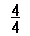x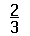=Select the denominator of the first fraction (3) and multiply the top and bottom of the second fraction (1/4) by that number: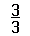x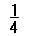=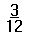These two fractions (8/12 and 3/12) have common denominators - the number 12 on the bottom of the fraction.

Subtract, using these two new fractions:-=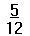Example 2: Say you have the fractions 3/5 and 2/7
Select the denominator of the second fraction (7) and multiply the top and bottom of the first fraction (3/5) by that number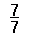x=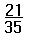Select the denominator of the first fraction (5) and multiply the top and bottom of the second fraction (2/7) by that numberx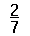=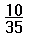These two fractions (21/35 and 10/35) have common denominators -- the number 35 on the bottom of the fraction.

We can now subtract, because the two new fractions have a common denominator:-=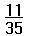Got it? Great! Then go to the SuperKids Math Worksheet Creator for Basic Fractions, and give it a try!Questions or comments regarding this site? webmaster@superkids.com